# Past Year Questions: Quality & Characteristics of Waste Water Notes | Study Topic wise GATE Past Year Papers for Civil Engineering - Civil Engineering (CE)

## Civil Engineering (CE): Past Year Questions: Quality & Characteristics of Waste Water Notes | Study Topic wise GATE Past Year Papers for Civil Engineering - Civil Engineering (CE)

The document Past Year Questions: Quality & Characteristics of Waste Water Notes | Study Topic wise GATE Past Year Papers for Civil Engineering - Civil Engineering (CE) is a part of the Civil Engineering (CE) Course Topic wise GATE Past Year Papers for Civil Engineering.
All you need of Civil Engineering (CE) at this link: Civil Engineering (CE)

Q. 1 The ultimate BOD (L0) a wastewater sample is estimated as 87% of COD. The COD of this wastewater is 300 mg/L. Considering first order BOD reaction rate constant k (use natural log) = 0.23 per day and temperature coefficient θ = 1.047, the BOD value (in mg/L, up to one decimal place) after three days of incubation at 2 7 °C for the w astew ater will b e _______    [2018 : 2 Marks, Set-I]
Ans: 160.226 mg/l

Ultimate BOD = 0.87
COD = 0.87 x 300 = 261 mg/l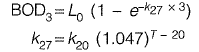For municipal sewage, at standard temperature, value of k((base e) = 0.23 per day. Thus, value 0.23 per day given is w.r.t. the standard temperature of 20°C.

= 0.23 (1,047)27-20

= 0.317 days-1
BOD3 = 261 (1 - e-0.317 x 3)
= 160.226 mg/l

Q. 2 For a given water sample, the ratio between BOD5-day, 2o°c and the ultimate BOD is 0.68. The value of the reaction rate constant k (on base e) (in day-1, up to two decimal places ) is [2017 : 2 Marks, Set-II]
Ans: 0.23 day-1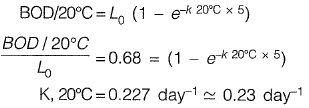Q. 3: Two wastewater streams A and B, having an indentical ultimate BOD are getting mixed to form the stream C. The temperature of the stream /A is 20°C and the temperature of the stream C is 10°C. It is given that

• the 5-day BOD of the stream A measured at 20°C = 50 mg/l
• BOD rate constant (base 10) at 20°C = 0.115 per day
• temperature coefficient = 1.135

The 5-day BOD (in mg/l, up to one decimal place) of the stream C, calculated at 10°C , is    [2017 : 2 Marks, Set-I]

Ans: 21.21mg/l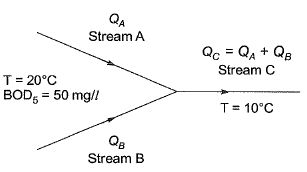For stream A,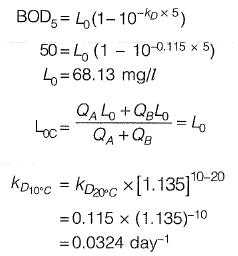For stream C,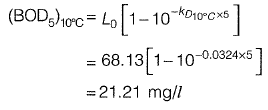Q. 4 For a wastewater sample, the three-day biochemical oxygen demand at incubation temperature of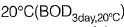is estimated as 200 mg/l. Taking the value of the first order BOD reaction rate constant as 0.22 day-1, the five-day BOD (expressed in mg/l) of the wastewater at incubation temperature of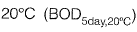would be______ .     [2016 : 2 Marks, Set-Il]
Ans: 276.19 mg/l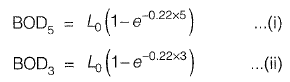From equation (i) and (ii), we get,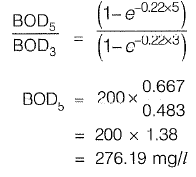Q. 5 : The 2-day and 4-day BOD values of a sewage sample are 100 mg/l and 155 mg/l, respectively. The value of BOD rate constant (expressed in per day) is________ .   [2016 : 2 Marks, Set-I]
Ans: 0.299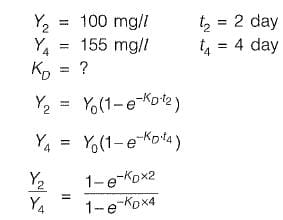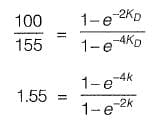1.55 - 1,55x = 1 - x2
x2 - 1.55x + 0.55= 0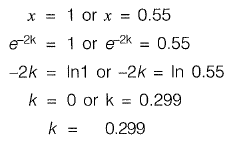Q. 6: Ultimate BOD of a river water sample is 20 mg/L. BOD rate constant (natural log) is 0.15 day-1. The respective values of BOD (in %) exerted and remaining after 7 days are:    [2015 : 2 Marks, Set-II]
(a) 45 and 55
(b) 55 and 45
(c) 65 and 35
(d) 75 and 25
Ans: (c)

BOD remaining after 7 days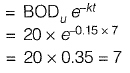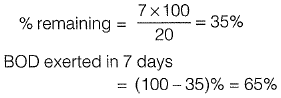The document Past Year Questions: Quality & Characteristics of Waste Water Notes | Study Topic wise GATE Past Year Papers for Civil Engineering - Civil Engineering (CE) is a part of the Civil Engineering (CE) Course Topic wise GATE Past Year Papers for Civil Engineering.
All you need of Civil Engineering (CE) at this link: Civil Engineering (CE)Use Code STAYHOME200 and get INR 200 additional OFF

## Topic wise GATE Past Year Papers for Civil Engineering

67 docs

Track your progress, build streaks, highlight & save important lessons and more!

,

,

,

,

,

,

,

,

,

,

,

,

,

,

,

,

,

,

,

,

,

;